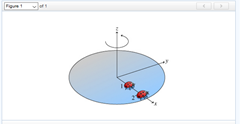# Problem: Two ladybugs sit on a rotating disk, as shown in the figure (the ladybugs are at rest with respect to the surface of the disk and do not slip). (Figure 1) Ladybug 1 is halfway between ladybug 2 and the axis of rotation.What is the ratio of the magnitude of the radial acceleration of ladybug 2 to that of ladybug 1?

###### FREE Expert Solution

$\overline{){{\mathbf{a}}}_{{\mathbf{c}}}{\mathbf{=}}\frac{{\mathbf{v}}^{\mathbf{2}}}{\mathbf{r}}{\mathbf{=}}{\mathbf{r}}{{\mathbf{\omega }}}^{{\mathbf{2}}}}$

We have seen that both ladybugs have equal angular velocity.

80% (351 ratings)###### Problem Details

Two ladybugs sit on a rotating disk, as shown in the figure (the ladybugs are at rest with respect to the surface of the disk and do not slip). (Figure 1) Ladybug 1 is halfway between ladybug 2 and the axis of rotation.What is the ratio of the magnitude of the radial acceleration of ladybug 2 to that of ladybug 1?